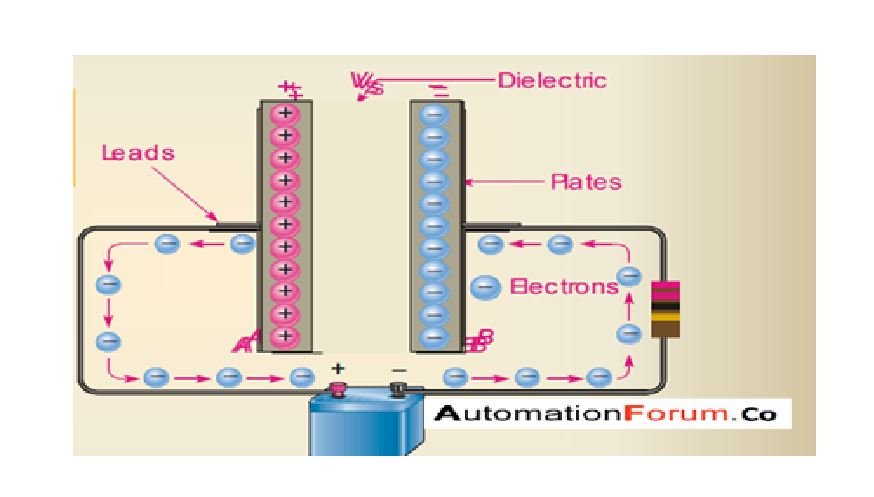# Types of capacitors and how can it be effectively used### What is a capacitor

A capacitor is an electrical component which has the ability to store charge. It consists of two parallel metal plates which are usually made up of aluminum and in between them an insulator is used as a dielectric, which can separate the two plates electrostatically from each other

Charging of a capacitor

### What is the capacitance of a capacitor

Capacitance can be considered as the measurement of the extent to which a capacitor can store charge, it is the ability of the capacitor to store charge

Capacitance can be defined as the charge on the plates of the capacitor per unit voltage across the plates of a capacitor

### Factors which influence the capacitance of a capacitor

• Distance of plate
• Area of overlap
• The permittivity of the dielectric

### How can we use a capacitor

• It can be used as temporary storage of DC electric charge almost like a battery
• Timing element in the DC circuit
• Phase-shifting element in AC circuit
• It can be used as an RMS voltage support element in an AC circuit
• It can be used as an AC circuit filter element

### Types of capacitors

Capacitors are used in electric circuits for various purposes different types of capacitors uses different types of dielectrics and the choice of the dielectric mostly depends upon the value of the capacitance and the stability required for the capacitor

Classification of capacitors

• Electrostatic capacitors
• Electrolytic capacitors
• Electrochemical capacitors
• Air capacitors
• Variable capacitors

### Electrolytic capacitors

These capacitors have capacitance up to 10000 micro-farads and they are compact because they use a very thin layer of dielectric and it can withstand very high-voltage without breaking down. Electrolytic capacitors have dedicated terminals and reversing the terminal can cause breakdown

It has two primary losses by resistance a series resistance due to the resistance of the electrolyte and a parallel resistance due to the leakage and polarization loss of the thin oxide layer

• Due to its higher capacitor strength, it is suitable for obstruction, decoupling, and filtering
• It can be used when larger capacitance value is required
• Due to its large capacitance, it can be used in DC supplies
• Ripple voltages will be reduced by this

### Air capacitors

These capacitors are used in radio tuning circuits air is used as a dielectric in variable capacitors, it consists of two sets of parallel one which is fixed and the other can be rotated on a spindle by changing the area of overlap between the plates could vary the capacitance of a capacitor

### Electrochemical capacitors

Electrochemical capacitors have higher capacitance compared to conventional capacitors. The difference between the conventional capacitor and an electrochemical capacitor is the surface area which is higher in the electrochemical capacitor they have a porous electrode structure and the charge separation distance is smaller than the other capacitors because it does a mechanism called the electric double layer

It has higher efficiency and longer life time and it is because of the high electrolytic conductivity and its ability to store charges

Electro-chemical capacitors can replace batteries. Electric double-layer it is the formation of two layers of opposite charge at the interface between the electrode and electrolyte one in the electrode and the other in the electrolyte

• Double layer has a separation distance in the nanometer range
• Smaller charge separation distance have given rise to higher capacitance

### Variable capacitors

These capacitors has small capacitance value and it is adjusted manually. A solid state device which is used as a variable capacitor is varactor diode and it is adjusted with electric signal. By using this capacitor the capacitance can be changed mechanically. it can be used to set the frequency resonance in LC circuit

### Electrostatic capacitors (conventional capacitors)

It is made up of two metal electrodes separated by a dielectric, dielectric is a non-conducting material which is inserted between the parallel plates. No chemical reaction is used to store charges. Electrons from one metal plate is transferred to the other so one side of the metal plates become deficient in electrons while the other has high electron and it would result an electric field across the dielectric and produce a voltage between the plates